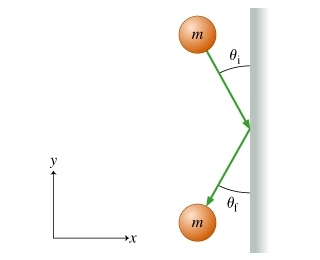# Problem: A ball of mass m moving with velocity v⃗ i strikes a vertical wall as shown in (Figure 1) . The angle between the ball's initial velocity vector and the wall is θi as shown on the diagram, which depicts the situation as seen from above. The duration of the collision between the ball and the wall is Δt, and this collision is completely elastic. Friction is negligible, so the ball does not start spinning. In this idealized collision, the force exerted on the ball by the wall is parallel to the x axis.What is the magnitude F of the average force exerted on the ball by the wall?Express your answer in terms of variables given in the problem.

###### FREE Expert Solution

Momentum:

$\overline{){\mathbf{p}}{\mathbf{=}}{\mathbf{m}}{\mathbf{v}}}$

Impulsive force:

$\overline{){\mathbf{F}}{\mathbf{=}}\frac{\mathbf{∆}\mathbf{p}}{\mathbf{∆}\mathbf{t}}}$

The y-component of the velocity does not change. Hence, no change in momentum occurs in the y-direction.

The x-component of the initial velocity is:###### Problem Details

A ball of mass m moving with velocity vi strikes a vertical wall as shown in (Figure 1) . The angle between the ball's initial velocity vector and the wall is θi as shown on the diagram, which depicts the situation as seen from above. The duration of the collision between the ball and the wall is Δt, and this collision is completely elastic. Friction is negligible, so the ball does not start spinning. In this idealized collision, the force exerted on the ball by the wall is parallel to the x axis.What is the magnitude F of the average force exerted on the ball by the wall?